# 12.5 Conic sections in polar coordinates  (Page 5/8)

 Page 5 / 8

For the following exercises, convert the polar equation of a conic section to a rectangular equation.

$25{x}^{2}+16{y}^{2}-12y-4=0$

$21{x}^{2}-4{y}^{2}-30x+9=0$

$64{y}^{2}=48x+9$

$96{y}^{2}-25{x}^{2}+110y+25=0$

$3{x}^{2}+4{y}^{2}-2x-1=0$

$5{x}^{2}+9{y}^{2}-24x-36=0$

For the following exercises, graph the given conic section. If it is a parabola, label the vertex, focus, and directrix. If it is an ellipse, label the vertices and foci. If it is a hyperbola, label the vertices and foci.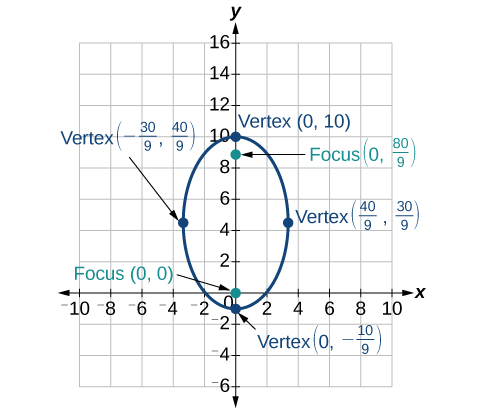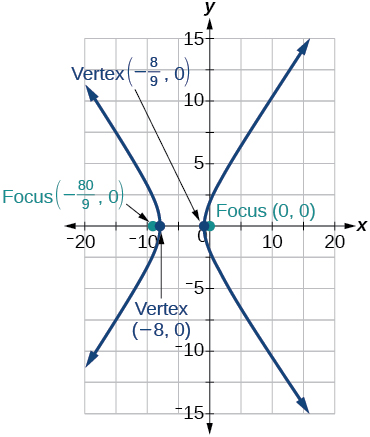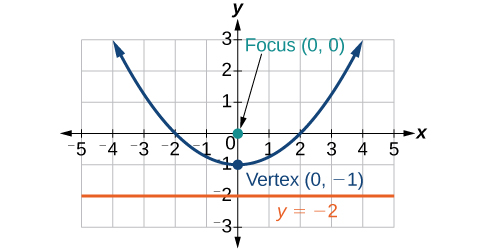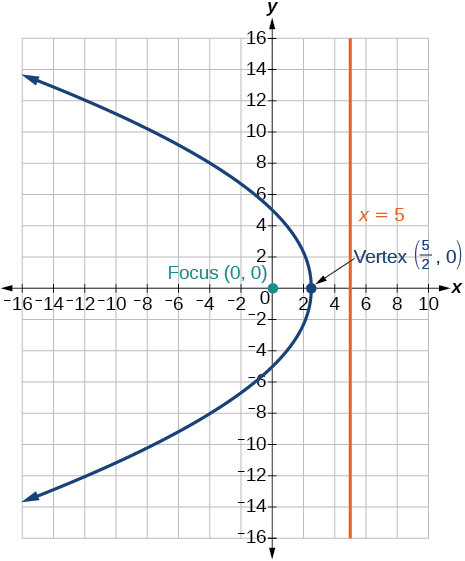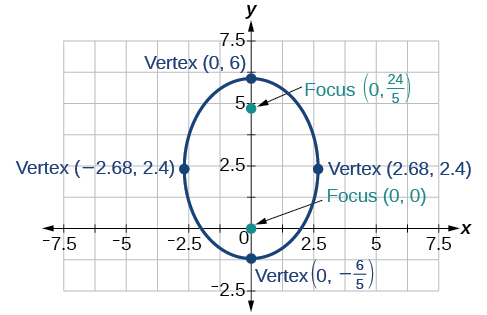For the following exercises, find the polar equation of the conic with focus at the origin and the given eccentricity and directrix.

Directrix: $x=4;\text{\hspace{0.17em}}e=\frac{1}{5}$

$r=\frac{4}{5+\mathrm{cos}\theta }$

Directrix: $x=-4;\text{\hspace{0.17em}}e=5$

Directrix: $y=2;\text{\hspace{0.17em}}e=2$

$r=\frac{4}{1+2\mathrm{sin}\theta }$

Directrix: $y=-2;\text{\hspace{0.17em}}e=\frac{1}{2}$

Directrix: $x=1;\text{\hspace{0.17em}}e=1$

$r=\frac{1}{1+\mathrm{cos}\theta }$

Directrix: $x=-1;\text{\hspace{0.17em}}e=1$

Directrix: $x=-\frac{1}{4};\text{\hspace{0.17em}}e=\frac{7}{2}$

$r=\frac{7}{8-28\mathrm{cos}\theta }$

Directrix: $y=\frac{2}{5};\text{\hspace{0.17em}}e=\frac{7}{2}$

Directrix: $y=4;\text{\hspace{0.17em}}e=\frac{3}{2}$

$r=\frac{12}{2+3\mathrm{sin}\theta }$

Directrix: $x=-2;\text{\hspace{0.17em}}e=\frac{8}{3}$

Directrix: $x=-5;\text{\hspace{0.17em}}e=\frac{3}{4}$

$r=\frac{15}{4-3\mathrm{cos}\theta }$

Directrix: $y=2;\text{\hspace{0.17em}}e=2.5$

Directrix: $x=-3;\text{\hspace{0.17em}}e=\frac{1}{3}$

$r=\frac{3}{3-3\mathrm{cos}\theta }$

## Extensions

Recall from Rotation of Axes that equations of conics with an $\text{\hspace{0.17em}}xy\text{\hspace{0.17em}}$ term have rotated graphs. For the following exercises, express each equation in polar form with $\text{\hspace{0.17em}}r\text{\hspace{0.17em}}$ as a function of $\text{\hspace{0.17em}}\theta .$

$xy=2$

${x}^{2}+xy+{y}^{2}=4$

$r=±\frac{2}{\sqrt{1+\mathrm{sin}\theta \mathrm{cos}\theta }}$

$2{x}^{2}+4xy+2{y}^{2}=9$

$16{x}^{2}+24xy+9{y}^{2}=4$

$r=±\frac{2}{4\mathrm{cos}\theta +3\mathrm{sin}\theta }$

$2xy+y=1$

## The Ellipse

For the following exercises, write the equation of the ellipse in standard form. Then identify the center, vertices, and foci.

$\frac{{x}^{2}}{25}+\frac{{y}^{2}}{64}=1$

$\frac{{x}^{2}}{{5}^{2}}+\frac{{y}^{2}}{{8}^{2}}=1;\text{\hspace{0.17em}}$ center: $\text{\hspace{0.17em}}\left(0,0\right);\text{\hspace{0.17em}}$ vertices: $\text{\hspace{0.17em}}\left(5,0\right),\left(-5,0\right),\left(0,8\right),\left(0,-8\right);\text{\hspace{0.17em}}$ foci: $\text{\hspace{0.17em}}\left(0,\sqrt{39}\right),\left(0,-\sqrt{39}\right)$

$\frac{{\left(x-2\right)}^{2}}{100}+\frac{{\left(y+3\right)}^{2}}{36}=1$

$9{x}^{2}+{y}^{2}+54x-4y+76=0$

$\frac{{\left(x+3\right)}^{2}}{{1}^{2}}+\frac{{\left(y-2\right)}^{2}}{{3}^{2}}=1\text{\hspace{0.17em}}\text{\hspace{0.17em}}\left(-3,2\right);\text{\hspace{0.17em}}\text{\hspace{0.17em}}\left(-2,2\right),\left(-4,2\right),\left(-3,5\right),\left(-3,-1\right);\text{\hspace{0.17em}}\text{\hspace{0.17em}}\left(-3,2+2\sqrt{2}\right),\left(-3,2-2\sqrt{2}\right)$

$9{x}^{2}+36{y}^{2}-36x+72y+36=0$

For the following exercises, graph the ellipse, noting center, vertices, and foci.

$\frac{{x}^{2}}{36}+\frac{{y}^{2}}{9}=1$

center: $\text{\hspace{0.17em}}\left(0,0\right);\text{\hspace{0.17em}}$ vertices: $\text{\hspace{0.17em}}\left(6,0\right),\left(-6,0\right),\left(0,3\right),\left(0,-3\right);\text{\hspace{0.17em}}$ foci: $\text{\hspace{0.17em}}\left(3\sqrt{3},0\right),\left(-3\sqrt{3},0\right)$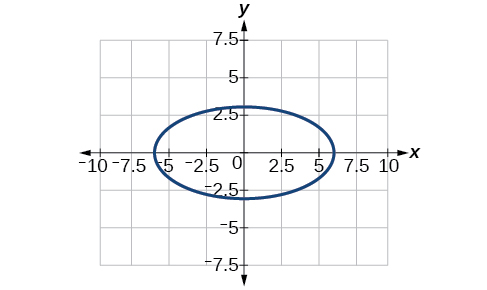$\frac{{\left(x-4\right)}^{2}}{25}+\frac{{\left(y+3\right)}^{2}}{49}=1$

$4{x}^{2}+{y}^{2}+16x+4y-44=0$

center: $\text{\hspace{0.17em}}\left(-2,-2\right);\text{\hspace{0.17em}}$ vertices: $\text{\hspace{0.17em}}\left(2,-2\right),\left(-6,-2\right),\left(-2,6\right),\left(-2,-10\right);\text{\hspace{0.17em}}$ foci: $\text{\hspace{0.17em}}\left(-2,-2+4\sqrt{3},\right),\left(-2,-2-4\sqrt{3}\right)$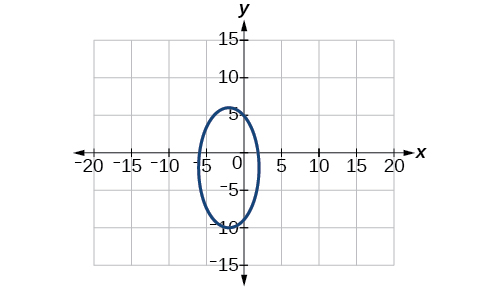$\text{\hspace{0.17em}}2{x}^{2}+3{y}^{2}-20x+12y+38=0$

For the following exercises, use the given information to find the equation for the ellipse.

Center at $\text{\hspace{0.17em}}\left(0,0\right),$ focus at $\text{\hspace{0.17em}}\left(3,0\right),$ vertex at $\text{\hspace{0.17em}}\left(-5,0\right)$

$\frac{{x}^{2}}{25}+\frac{{y}^{2}}{16}=1$

Center at $\text{\hspace{0.17em}}\left(2,-2\right),$ vertex at $\text{\hspace{0.17em}}\left(7,-2\right),$ focus at $\text{\hspace{0.17em}}\left(4,-2\right)$

A whispering gallery is to be constructed such that the foci are located 35 feet from the center. If the length of the gallery is to be 100 feet, what should the height of the ceiling be?

Approximately 35.71 feet

## The Hyperbola

For the following exercises, write the equation of the hyperbola in standard form. Then give the center, vertices, and foci.

$\frac{{x}^{2}}{81}-\frac{{y}^{2}}{9}=1$

$\frac{{\left(y+1\right)}^{2}}{16}-\frac{{\left(x-4\right)}^{2}}{36}=1$

$\frac{{\left(y+1\right)}^{2}}{{4}^{2}}-\frac{{\left(x-4\right)}^{2}}{{6}^{2}}=1;\text{\hspace{0.17em}}$ center: $\text{\hspace{0.17em}}\left(4,-1\right);\text{\hspace{0.17em}}$ vertices: $\text{\hspace{0.17em}}\left(4,3\right),\left(4,-5\right);\text{\hspace{0.17em}}$ foci: $\text{\hspace{0.17em}}\left(4,-1+2\sqrt{13}\right),\left(4,-1-2\sqrt{13}\right)$

$9{y}^{2}-4{x}^{2}+54y-16x+29=0$

$3{x}^{2}-{y}^{2}-12x-6y-9=0$

$\frac{{\left(x-2\right)}^{2}}{{2}^{2}}-\frac{{\left(y+3\right)}^{2}}{{\left(2\sqrt{3}\right)}^{2}}=1;\text{\hspace{0.17em}}$ center: $\text{\hspace{0.17em}}\left(2,-3\right);\text{\hspace{0.17em}}$ vertices: $\text{\hspace{0.17em}}\left(4,-3\right),\left(0,-3\right);\text{\hspace{0.17em}}$ foci: $\text{\hspace{0.17em}}\left(6,-3\right),\left(-2,-3\right)$

For the following exercises, graph the hyperbola, labeling vertices and foci.

root under 3-root under 2 by 5 y square
The sum of the first n terms of a certain series is 2^n-1, Show that , this series is Geometric and Find the formula of the n^th
cosA\1+sinA=secA-tanA
why two x + seven is equal to nineteen.
The numbers cannot be combined with the x
Othman
2x + 7 =19
humberto
2x +7=19. 2x=19 - 7 2x=12 x=6
Yvonne
because x is 6
SAIDI
what is the best practice that will address the issue on this topic? anyone who can help me. i'm working on my action research.
simplify each radical by removing as many factors as possible (a) √75
how is infinity bidder from undefined?
what is the value of x in 4x-2+3
give the complete question
Shanky
4x=3-2 4x=1 x=1+4 x=5 5x
Olaiya
hi can you give another equation I'd like to solve it
Daniel
what is the value of x in 4x-2+3
Olaiya
if 4x-2+3 = 0 then 4x = 2-3 4x = -1 x = -(1÷4) is the answer.
Jacob
4x-2+3 4x=-3+2 4×=-1 4×/4=-1/4
LUTHO
then x=-1/4
LUTHO
4x-2+3 4x=-3+2 4x=-1 4x÷4=-1÷4 x=-1÷4
LUTHO
A research student is working with a culture of bacteria that doubles in size every twenty minutes. The initial population count was  1350  bacteria. Rounding to five significant digits, write an exponential equation representing this situation. To the nearest whole number, what is the population size after  3  hours?
v=lbh calculate the volume if i.l=5cm, b=2cm ,h=3cm
Need help with math
Peya
can you help me on this topic of Geometry if l help you
litshani
( cosec Q _ cot Q ) whole spuare = 1_cosQ / 1+cosQ
A guy wire for a suspension bridge runs from the ground diagonally to the top of the closest pylon to make a triangle. We can use the Pythagorean Theorem to find the length of guy wire needed. The square of the distance between the wire on the ground and the pylon on the ground is 90,000 feet. The square of the height of the pylon is 160,000 feet. So, the length of the guy wire can be found by evaluating √(90000+160000). What is the length of the guy wire?
the indicated sum of a sequence is known as
how do I attempted a trig number as a starter
cos 18 ____ sin 72 evaluateByByBy Jill ZerressenBy Hope PercleBy Marion CabalfinBy OpenStaxBy Mary MateraBy OpenStaxBy OpenStaxBy Christine ZeelieBy Kevin AmaratungaBy Rhodes